# 一、类的6个默认成员函数

class Date {};


如果一个类中什么成员都没有，简称为空类。空类中什么都没有吗？并不是的，任何一个类在我们不写的情况下，都会自动生成下面 6 个默认成员函数。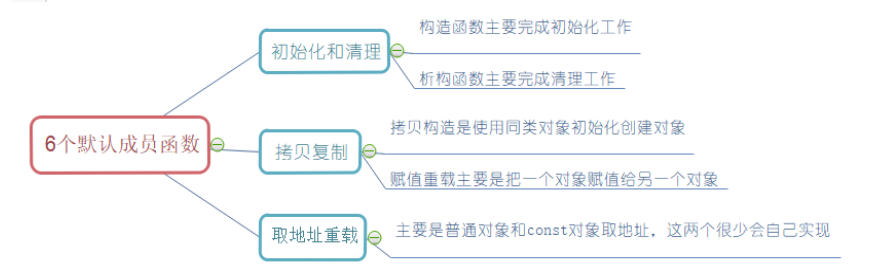# 二、构造函数

概念

场景


class Date
{
public :
void SetDate(int year, int month, int day){
_year = year;
_month = month;
_day = day;
}
void Display(){

cout << _year << " "<<_month<<" "<<_day<< endl;
}
private:
int _year;
int _month;
int _day;

};
int main(){
Date d1;
d1.SetDate(2020, 3, 17);
d1.Display;

Date d2;
d2.SetDate(2020, 3, 18);
d2.Display;
return 0;
}


class Date
{
public :
//1.无参构造函数
Date()
{

}
//2.带参构造函数
Date(int year, int month, int day){
_year = year;
_month = month;
_day = day;
}
void Display(){

cout << _year << " "<<_month<<" "<<_day<< endl;
}
private:
int _year;
int _month;
int _day;

};
int main(){
Date d1; //调用无参构造函数
Date d2(2022,3,17);//调用带参构造函数

// 注意：如果通过无参构造函数创建对象时，对象后面不用跟括号，否则就成了函数声明
// 以下代码的函数：声明了d3函数，该函数无参，返回一个日期类型的对象
Date d3();

return 0;
}

1. 函数名与类名相同。

2.无返回值。

3.对象实例化时编译器自动调用对应的构造函数。

4.构造函数可以重载。

1.如果类中没有显式定义构造函数，则C++编译器会自动生成一个无参的默认构造函数，一旦用户显式定义编译器将不再生成 。

class Date
{
public:
/*如果用户显示定义构造函数，编译器将不再生成默认构造函数。
Date(int year,int month,int day){

_year =year;
_month= month;
_day  = day;
} */
//如果没有显示定义构造函数，对象也能创建成功，此时调用的是编译器创建的构造函数。
private:
int _year;
int _month;
int _day;
};

int main(){
Date d;

return 0;
}

C++ 把我们的变量分成内置类型 (int、char、指针 …) 和自定义类型 (struct/class)

1、内置类型不会初始化
2、自定义类型它会调用它的无参构造函数初始化

2.关于编译器生成的默认构造函数，很多童鞋会有疑惑：在我们不实现构造函数的情况下，编译器会生成默认构造函数，但看起来默认构造函数又没什么用 。

C++ 把类型分成内置类型 (基本类型) 和自定义类型，这里只是说它不会处理内置类型，而自定义类型它会去调用它的无参构造函数来初始化。

class Time{
public:
Time(){                   // 默认构造函数
cout << "Time()" << endl;
_hour = 0;
_minute = 0;
_second = 0;
}
private:
int _hour;
int _minute;
int _second;
};
class Date
{
private:
// 内之类型
int _year;
int _month;
int _day;
// 自定义类型
Time _t;
};
int main(){

Date d;
return 0;
}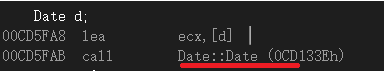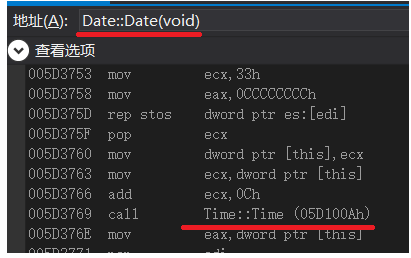3.到了 C++11 时 C++ 针对这里的情况做了补充 ？

C++11 时，语法委员会针对这里打了一个补丁，也就是在变量声明中加上缺省值。

class A
{
public:
A()
{
cout << "A()" << endl;
}
private:
int _a1;
int _a2;
};
class Date
{
public:
Date()
{
_year = 2022;//只初始化_year，其它默认
}
void Print()
{
cout << _year << "/" << _month << "/" << _day << endl;
}
private:
//C++11 缺省值
int _year = 0;
int _month = 1;
int _day = 1;
A _a;
};
int main()
{
Date d1;
d1.Print();

return 0;
}


4.无参的构造函数和全缺省的构造函数都称为默认构造函数，并且默认构造函数只能有一个。

class Date
{
public:
Date()       // 无参构造函数 或 全缺省构造函数 都称为 默认构造函数
{
_year = 0;
_month = 1;
_day = 1;
}            //全缺省构造函数
Date(int year = 1, int month = 1, int day = 1)
{
_year = year;
_month = month;
_day = day;
}
void Print()
{
cout << _year << "/" << _month << "/" << _day << endl;
}
private:
int _year;
int _month;
int _day;
};
int main()
{
Date d1;  //  此时调用构造函数会出现歧义，不知道调用那个
Date d2(3);// 此时调用构造函数会出现歧义，不知道调用那个
return 0;
}


5.成员变量的命名风格

class Date
{
public:
Date(int year)//不好的命名风格
{
year = year;
}
Date(int year,int month)//好的命名风格
{
_year = year;
_month = month;
}
private:
int year;
int _year;
int _month;
};
int main()
{
Date d1(1);
Date d2(1, 2);
return 0;
}


NO ~~，实际上在 Date 里它会采用就进原则，也可以认为是局部优先。

# 三、析构函数

class Date
{
public:
void Print()
{
cout << _year << "/" << _month << "/" << _day << endl;
}
~Date()
{
cout << "~Date()" << endl;//证明调用了析构函数
}
private:
int _year;
int _month;
int _day;
};
int main()
{
Date d1;
return 0;
}


特性:

1. 析构函数名是在类名前加上字符 ~。

2. 无参数无返回值 (不支持重载)。因为没有参数

3. 一个类有且只有一个析构函数。若未显式定义，系统会自动生成默认的析构函数。

4. 对象生命周期结束时，C++ 编译系统系统自动调用析构函数。

对于类里有申请空间操作的，必须要自己写析构函数，要不然会出现内存泄露.

class Stack
{
public:
Stack(int capacity = 4)
{
_a = (int*)malloc(sizeof(int)*capacity);
if(_a == nullptr)
{
cout << "malloc fail" << endl;
exit(-1);
}
_top = 0;
_capacity = capacity;
}
~Stack()
{
free(_a);
_a = nullptr;
}
private:
int* _a;
int _top;
int _capacity;
};
int main()
{
Stack st;
return 0;
}


class Stack
{
public:
Stack(int capacity = 4)
{
_a = (int*)malloc(sizeof(int)*capacity);
if(_a == nullptr)
{
cout << "malloc fail" << endl;
exit(-1);
}
_top = 0;
_capacity = capacity;
}
private:
int* _a;
int _top;
int _capacity;
};
int main()
{
Stack st;
return 0;
}


1、内置类型成员不处理
2、自定义类型成员会去调用它的析构函数

class Time{
public:
Time(){
cout << "Time()" << endl;
_hour = 0;
_minute = 0;
_second = 0;
}
~Time(){   //写不写都可以
cout << "说明调用析构函数"<<endl;
}
private:
int _hour;
int _minute;
int _second;
};

class Date
{
public :
Date(int year, int month, int day){
_year = year;
_month = month;
_day = day;
}
~Date(){    //对于日期而言 ，写不写都可以

}
private:
// 内之类型
int _year;
int _month;
int _day;
// 自定义类型
Time _t;
};
int main(){

Date d(2020,3,17);
return 0;
}


对于类里面进行了空间申请的，必须调用析构函数进行空间的清理。析构函数必须自己写，如果只是用编译器默认给出的，就会出现内存泄露。

class Stack
{
public:
Stack(int capacity = 4)
{
_a = (int*)malloc(sizeof(int)*capacity);
if(_a == nullptr)
{
cout << "malloc fail" << endl;
exit(-1);
}
_top = 0;
_capacity = capacity;
}
~Stack()
{
free(_a);
_a = nullptr;
}
private:
int* _a;
int _top;
int _capacity;
};
class MyQueue
{
private:
Stack _pushST;
Stack _popST;
};
int main()
{
MyQueue mq;
return 0;
}


1、初始化 _pushST 和 _popST和销毁_pushST 和 _popST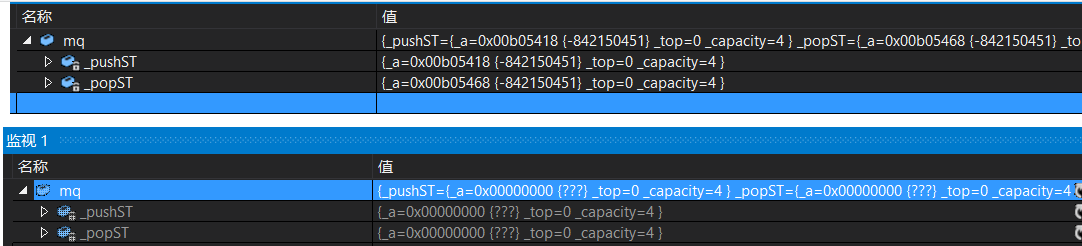# 四,拷贝函数

1.拷贝构造函数典型的调用场景

1：使用已存在的对象创建新对象

2：函数参数类型为类类型对象

3：函数返回值类型为类类型对象

class  Date
{
public :
Date(int year = 1900, int month=1, int day=1){    //构造函数
_year = year;
_month = month;
_day = day;
cout << "Date(int ,int ,int ):" << this << endl;
}
Date(const Date & d){                             //拷贝构造
_year = d._year;
_month = d._month;
_day = d._day;
cout << "Date(const Date & d) " << this<<endl;
}
~Date(){                                          //析构函数
cout << "~Date()" << this << endl;
}
private:
int _year;
int _month;
int _day;

};

// 函数形参为值类型，返回值也为值类型，传递实参为对象，返回类型也为对象类型
Date TestDate(Date param){
return param;
}

//函数形参为类类型引用 ，返回值类型为类类型引用。
const Date& TestDate(const Date & param){
return param;
}

void TestFunc(){
Date d1;
Date d2(d1);  //使用已存在的对象创建新对象
TestDate(d1); //两种情况
}

int main(){
TestFunc();
return 0;
}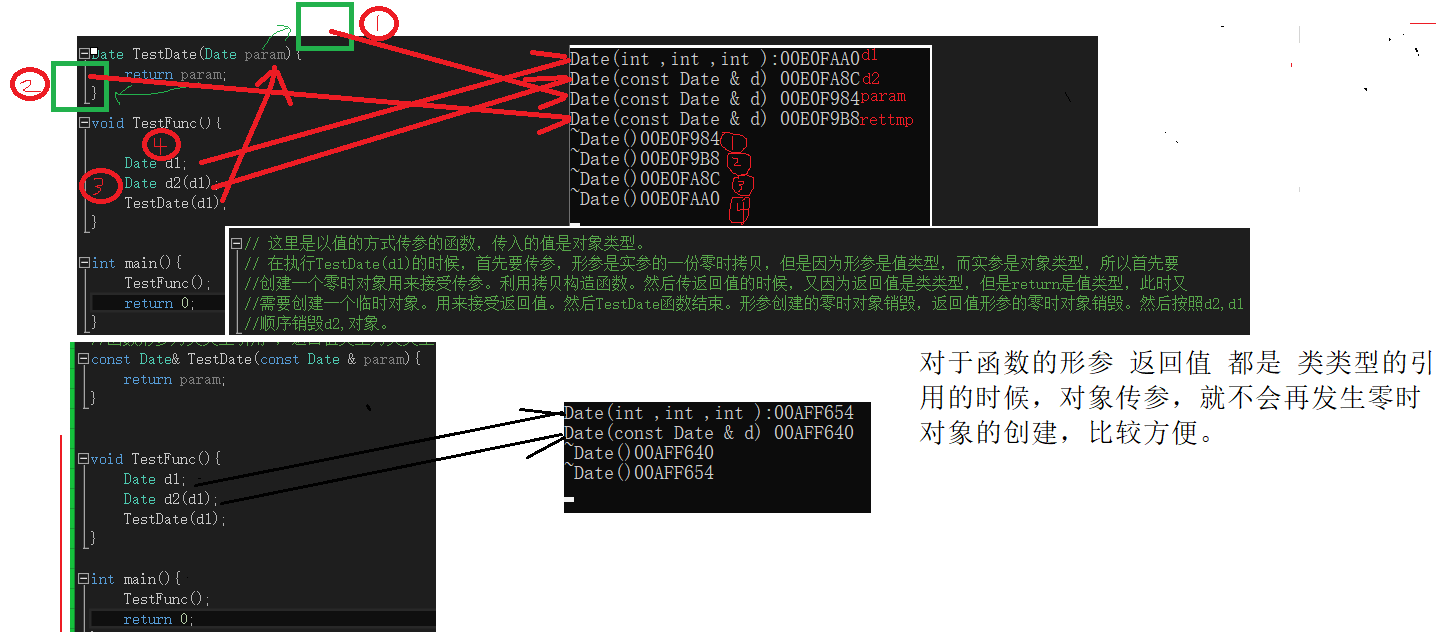class Date
{
public:
// 全缺省构造函数
Date(int year = 1, int month = 1, int day = 1)
{
_year = year;
_month = month;
_day = day;
}
//Date d3(d2);

//  传值传参拷贝构造 这种方法错误，不能实现。
Date(const Date d)
{
_year = d._year;
_month = d._month;
_day = d._day;
}
//Date d2(d1);

//  类类型对象的引用,但是不安全。
Date(Date & d)
{
_year = d._year;
_month = d._month;
_day = d._day;
_day++;
}
//Date d3(d2);

// 类类型对象的引用，加const更安全。
Date(const Date& d)
{
_year = d._year;
_month = d._month;
_day = d._day;
}
//Date d3(d2);

//指针类传参，也可以实现。
Date(const Date* p)
{
_year = p->_year;
_month = p->_month;
_day = p->_day;
}
void Print()
{
cout << _year << "/"<< _month << "/" << _day <<	endl;
}
private:
int _year;
int _month;
int _day;
};
int main()
{
int a =10 ;
int b =20;
int a =b; //这种方式可以实现拷贝
int a(b);//这种方式也可以实现拷贝

Date d1;
Date d2(d1);
d1.Print();
d2.Print();
//拷贝复制一个d2对象
Date d3(d2);
d3.Print();
//指针也可以，但不好
Date d4(&p2);
d4.Print();
return 0;
}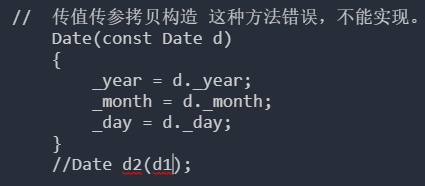error C2652：非法的复制构造函数，第一个参数不应是 “Date”。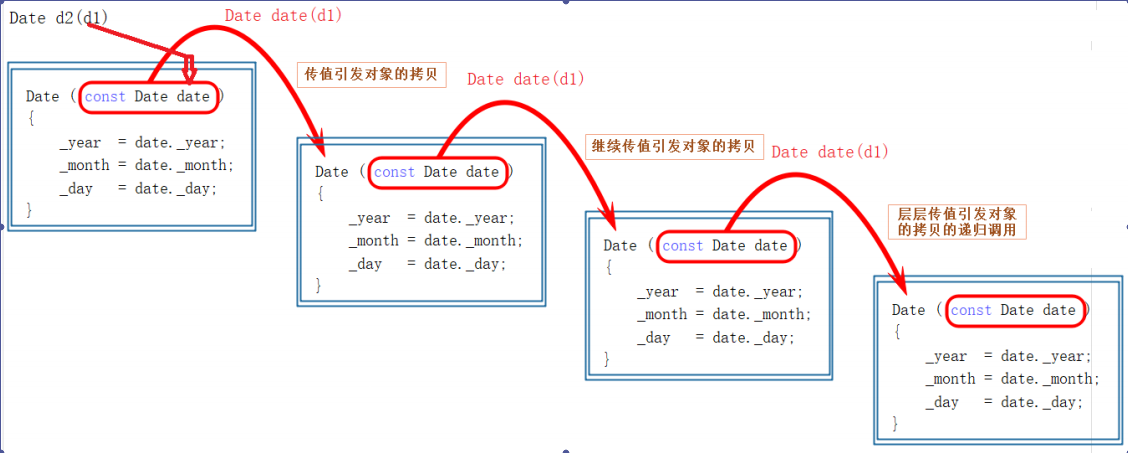说明：

如果拷贝函数参数是类类型的引用，那么将d1拿过来，拷贝d2.此时d 就是d1 的引用，所以将d类里面的值，传给d2(this指的就是d2),就是d1将自己的参传给d2.可以实现。

如果拷贝函数参数是值类型，形参是实参的一份拷贝。那么将d1传给值d,此时首先就要创建一个零时对象，用来接受d1。所以创建一个零时对象之后，又要调用拷贝构造，但是此时又要传参，传参又要创建一个零时对象，然后又要拷贝。所以会一直循换下去。

1、这里用引用解决了问题，因为形参是实参的别名，即 d是 d2 的别名

注意如果引用传参，不是做输出型参数，最好加 const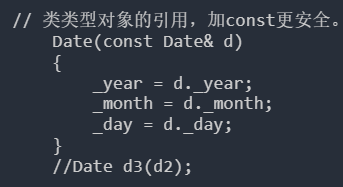2、当然也能使用指针解决，但并没有引用好用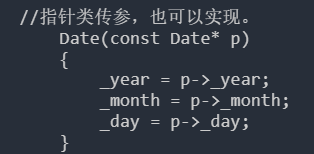特性

class Time
{
public:
Time(){        //Time构造函数
_hour = 0;
_minute = 0;
_second = 0;
cout <<"Time构造函数"<<endl;
}

Time(const Time & t){ // Time拷贝函数
_hour = t._hour;
_minute = t._minute;
_second = t._second;
cout <<"Time拷贝函数"<<endl;

}
private:
int _hour;
int _minute;
int _second;
};

class Date //没写拷贝函数和构造函数，但是对象还可以创建。编译器自己生成了构造函数和拷贝构造函数
{
private:
int _year = 2020;;
int _month =3;
int _day =7;
Time _t;
public:
void Display(){
cout << _year<<" "<<_month<<" "<<_day<<" "<< endl;
}
};

int main(){
Date d1;  //创建d1时，编译器会调用编译器生成的Date类的构造函数。然后_year,_month,_day都是他的默认值。然后到Time_t的时候，就会去调用Time类的 构造函数。

d1.Display(); // 显示 Time构造函数  2020 3 7

Date d2(d1);  //编译器会自动生成拷贝构造函数 。编译器给Date生成的拷贝构造函数，将d1._year _month _day 拷贝到d2放到了里面。然后调用Time类的构造函数 和拷贝构造函数 完成Date中_t 的拷贝。

d2.Display();  //  显示 Time构造函数 Time拷贝构造函数 2020 3 7

return 0;
}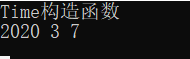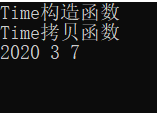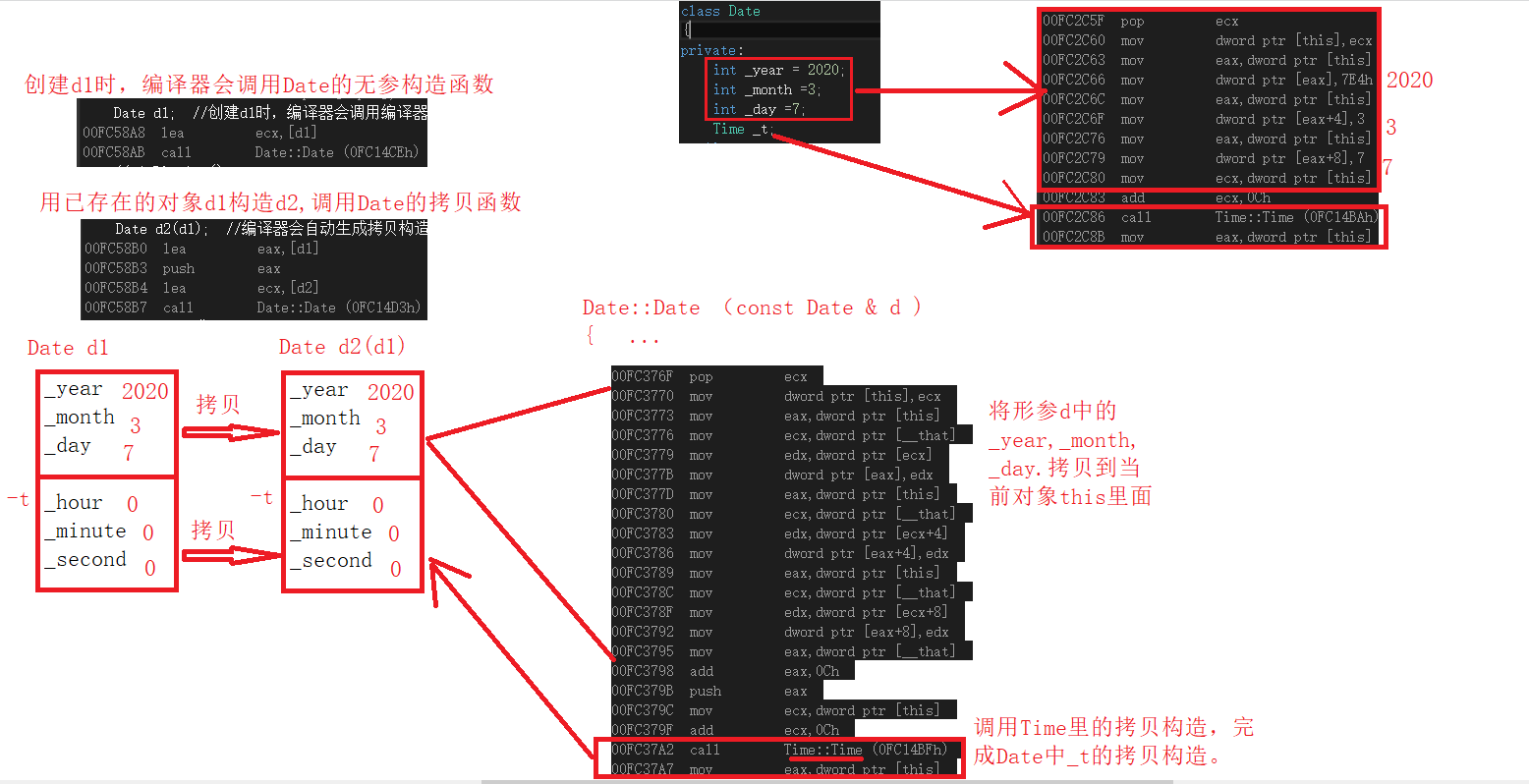1、内置类型，字节序的浅拷贝 (一个字节一个字节的拷贝)。

Date里的int _year int _month int_day 拷贝到 新的 Date对象里面。

2、自定义类型，会去调用它的拷贝构造完成拷贝。

Time拷贝函数。将Time 里面的_hour _minute _second 拷贝到 新的Time类对象里面。

class Stack
{
public:
Stack(int capacity = 4)
{
_a = (int*)malloc(sizeof(int)*capacity);
if(_a == nullptr)
{
cout << "malloc fail" << endl;
exit(-1);
}
_top = 0;
_capacity = capacity;
}
~Stack()
{
free(_a);
_a = nullptr;
}
private:
int* _a;
int _top;
int _capacity;
};
int main()
{
Stack st1;
Stack st2(st1);
return 0;
}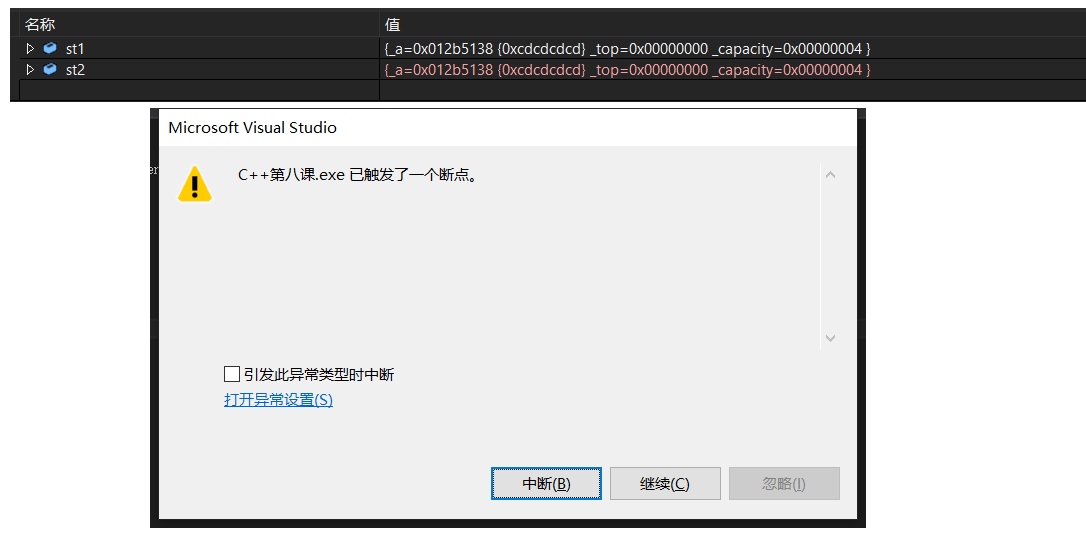此时 st1 和 st2 指向同一块空间，那么问题来了，谁先被析构呢 —— 根据栈的特性后进先出，所以这里先被析构的是 st2。st2 被析构后再析构 st1 时程序就崩溃了，因为同一块空间不能释放两次。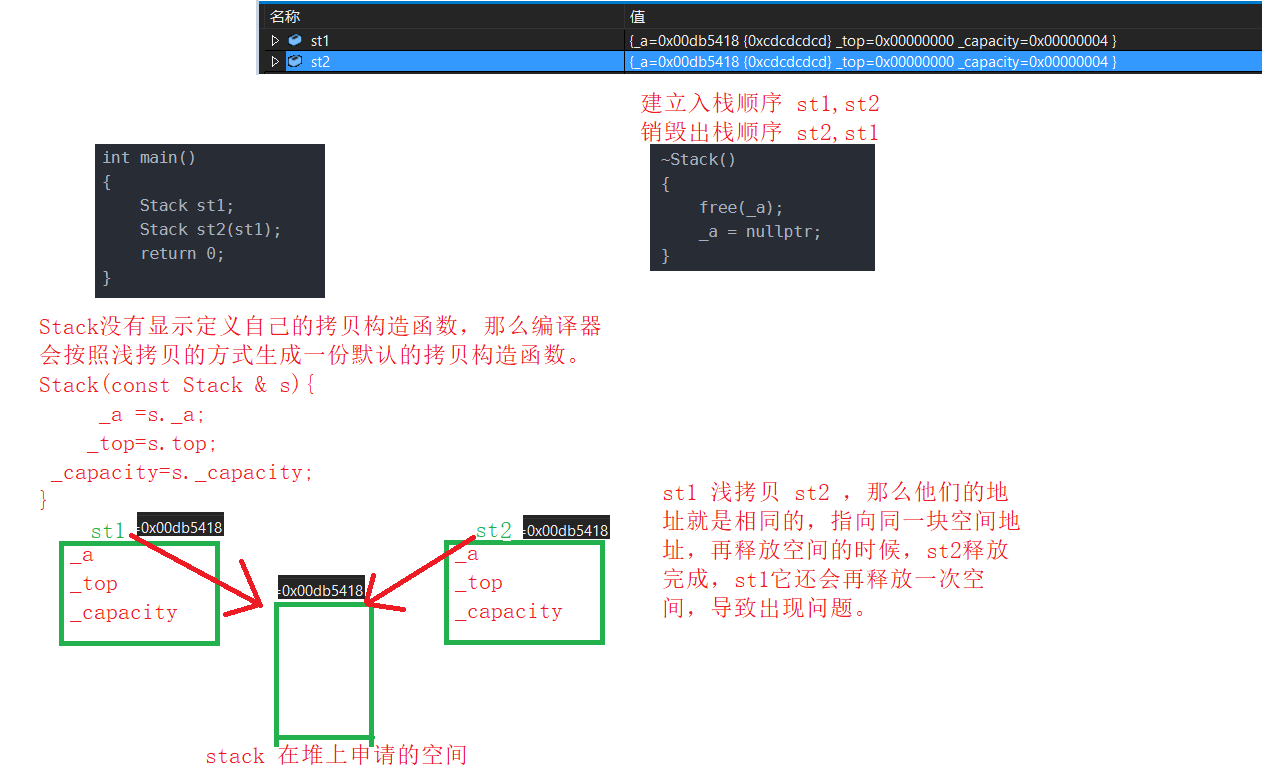拷贝 | 拷贝构造

int p()
{
int ret = 0;
return ret;
}
Date q()
{
Date ret;
return ret;
}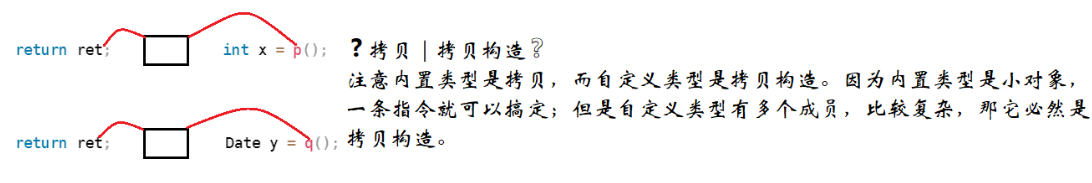# 五、赋值运算符重载 &&运算符重载

class Date
{
public:
Date(int year = 0, int month = 1, int day = 1)
{
_year = year;
_month = month;
_day = day;
}
private:
int _year;
int _month;
int _day;
};
int main()
{
Date d1(2021, 10, 11);
Date d2(2020, 11, 11);
Date d3(2021, 11, 11);

//d1 == d2;//错误

return 0;
}


d1 == d2 为啥错？

C++ 为了增强代码的可读性引入了运算符重载，运算符重载是具有特殊函数名的函数，函数里的实现就是行为。它也具有其返回值类型，函数名字以及参数列表，其返回值类型与参数列表与普通的函数类似。

class Date
{
public:
Date(int year = 0, int month = 1, int day = 1)
{
_year = year;
_month = month;
_day = day;
}
//private:
public://这里破坏封装使得可以在类外访问成员
int _year;
int _month;
int _day;
};
bool operator==(const Date& x1, const Date& x2)
{
return x1._year == x2._year
&& x1._month == x2._month
&& x1._day == x2._day;
}
int main()
{
Date d1(2021, 10, 11);
Date d2(2020, 11, 11);
Date d3(2021, 11, 11);

operator==(d1, d2);//可以这样调用，但是这样可读性很差，还不如写一个函数
d1 == d2;//同上，如果没有重载会报错，如果重载了它会转换为 operator==(d1, d2);
return 0;
}


说明:

class Date
{
public:
Date(int year = 0, int month = 1, int day = 1)
{
_year = year;
_month = month;
_day = day;
cout<<"Date(int,int,int)"<<this<<endl;
}

// 写一个比较函数。
bool IsEqual (const Date& d){
return  _year == d._year&&
_month == d._month&&
_day == d._day;

}
//作为全局函数 运算符重载，需要两个参数
bool operator==(const Date& x1, const Date& x2)//err
{
return x1._year == x2._year
&& x1._month == x2._month
&& x1._day == x2._day;
}

// 作为成员函数，只需要传入一个参数，因为他自己还有一个this指针
//bool operator==(/*Date* this*/, const Date& x)
bool operator==(const Date& x)
{
return _year == x._year
&& _month == x._month
&& _day == x._day;
}
private:
int _year;
int _month;
int _day;
};
int main()
{
int a =10;               //内置类型的比较
int b =20;
if (a<b){                //这个可以实现
cout <<"a<b"<<endl ;
}
else if (a>b){
cout <<"a>b"endl;
}
else{
cout <<"a==b"<<endl;
}

Date d1(2021, 10, 11);
Date d2(d1); //拷贝
if(d1==d2){   //很直观但是，编译器不知道类应该怎么比较，所以会报错。
//可以通过运算符重载，就可以直接使用这个。
}

if(d1.IsEqual(d2))     //调用一个我们写的比较函数，可以实现对象之间的比较。
{
cout<<"d1==d2"<<endl;
}

d1.operator==(d2); //这里实现了运算符重载可以这么写，也可以直接写下面的形式。
d1 == d2;//同上，编译器会自动识别转换为d1.operator==(d2); -> d1.operator(&d1, d2);
return 0;
}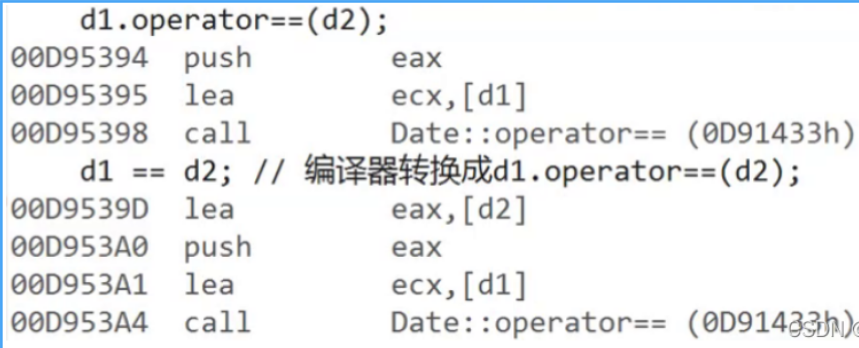照虎画猫写一个d1<d2

class Date
{
public:
Date(int year = 0, int month = 1, int day = 1)
{
_year = year;
_month = month;
_day = day;
}
bool operator<(const Date& x)//ok
{
if(_year < x._year)
return true;
else if(_year == x._year && _month < x._month)
return true;
else if(_year == x._year && _month == x._month && _day < x._day)
return true;
else
return false;
}
private:
int _year;
int _month;
int _day;
};
int main()
{
Date d1(2021, 10, 11);
Date d2(2020, 11, 11);
Date d3(2021, 11, 11);

cout << d1 < d2 << endl;//err
cout << (d1 < d2) << endl;//ok,d1.operator<(d2); -> d1.operator<(&d1, d2);
return 0;
}


注意:

1,不能通过连接其他符号来创建新的操作符：比如 operator@。

2,重载操作符必须有一个类类型或者枚举类型的操作数。

3,用于内置类型的操作符，其含义不能改变，例如：内置的整型+，不能改变其含义。

4,作为类成员的重载函数时，其形参看起来比操作数数目少 1 成员函数的操作符有一个默认的形参 this，限定为第一个形参。

5， .* 、:: 、sizeof 、?: 、. ，注意以上 5 个运算符不能重载。这个经常在笔试选择题中出现，注意 * 是可以重载的，这里不能重载的是 .*。

赋值运算符重载

class Date
{
public:
Date(int year = 0, int month = 1, int day = 1)
{
_year = year;
_month = month;
_day = day;
}
void operator=(const Date& x)
{
_year = x._year;
_month = x._month;
_day = x._day;
}
private:
int _year;
int _month;
int _day;
};
int main()
{
Date d1(2021, 10, 11);
Date d2(2020, 11, 11);
Date d3(2021, 11, 11);
//赋值运算符重载
d1 = d3;
//拷贝构造
Date d4(d3);

Date d5 = d3;//拷贝构造还是赋值运算符重载？？？ ———— 这里是拷贝构造，因为这里是在实例化对象，并且它同 Date d5(d3);
return 0;
}


说明:

对于上面写的赋值运算符重载写的不够好?

我们说我们去重载运算符，就是控制这个运算符的行为。

1、从赋值运算符的特性来说，它要能支持连续赋值 —— d1 = d2 = d3;。

int i = 1;
int j = 2;
int k = 3;
i = j = k;//连续赋值(从右至左),注意连续赋值是有返回值的。这里最后i去接收j=k的返回值


2、d1 = d1 自己赋值给自己，可以，但是没意义，还有可能会破坏之前的拷贝。所以赋值重载中为了防止这种情况它还要控制一下条件。

class Date
{
public:
Date(int year = 0, int month = 1, int day = 1)
{
_year = year;
_month = month;
_day = day;
}

// 用X 给 this 赋值，加const 保证了安全性，但是没有返回值不能满足 连续赋值。
void operator=(const Date& x)
{
_year = x._year;
_month = x._month;
_day = x._day;
}

//因为返回值是Date类型对象this指针。所以
//d1 = d2 = d3 时，d3赋值给d2，然后的d2赋值给d1.可以实现
// 用X 给 this 赋值，加const 保证了安全性
Date& operator=(const Date& x)
{
if(this != &x)
{
_year = x._year;
_month = x._month;
_day = x._day;
}

return *this;
}

private:
int _year;
int _month;
int _day;
};
int main()
{
Date d1(2022, 3, 21);
Date d2(2021, 3, 21);
Date d3(2022, 3, 21);

d1 = d2 = d3;

d1 = d1;
return 0;
}


说明:

同样如果我们不写赋值重载，编译器也会默认生成 .

1、内置类型成员会完成值拷贝。
2、自定义类型成员会去调用它的赋值重载。

class Time
{
public:
Time& operator=(const Time& _t)
{
cout << "Time& operator=(const Time& _t)" << endl;
return *this;
}
};
class Date
{
public:
Date(int year = 0, int month = 1, int day = 1)
{
_year = year;
_month = month;
_day = day;
}
void Print()
{
cout << _year << "/" << _month << "/" << _day << endl;
}
private:
int _year;
int _month;
int _day;

Time _t;
};
int main()
{
Date d1(2022, 3, 11);
d1.Print();
Date d2(2023, 3, 12);

d1 = d2; //用已存在的d2去构建d1,调用拷贝构造函数，对于_year _month _day 都直接拷贝
d1.Print();//对于Time_t 要去调用Time类型的拷贝构造函数。
return 0;
}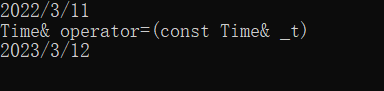### 六、日期类的实现

Date.h

#pragma once
#include<iostream>
#include<assert.h>
#include<stdbool.h>
using namespace std;
class Date
{
public:
//获取某年某月的天数
int GetMonthDay(int year, int month)
{
assert(month > 0 && month < 13);
//默认平年
int monthDays = { 0, 31, 28, 31, 30, 31, 30, 31, 31, 30, 31, 30, 31 };
//判断润年的二月
if (month == 2 && ((year % 4 == 0 && year % 100 != 0) || year % 400 == 0))
{
return 29;
}
return monthDays[month];
}
Date(int year = 0, int month = 1, int day = 1)
{
_year = year;
_month = month;
_day = day;
//判断日期是否合法
if (_year < 0 || _month <= 0 || _month >= 13 || _day <= 0 || _day > GetMonthDay(_year, _month))
{
cout << _year << "/" << _month << "/" << _day << "->";
cout << "非法日期" << endl;
}
}
void Print() const
{
cout << _year << "/" << _month << "/" << _day << endl;
}
bool operator>(const Date& d) const
{
if (_year > d._year)
return true;
else if (_year == d._year && _month > d._month)
return true;
else if (_year == d._year && _month == d._month && _day > d._day)
return true;
else
return false;
}
bool operator==(const Date& d) const
{
return _year == d._year && _month == d._month && _day == d._day;
}
bool operator>=(const Date& d) const
{
return *this > d || *this == d;//复用operator>、operator==
}
bool operator<(const Date& d) const
{
return !(*this >= d);//复用operator>=，再取反
}
bool operator<=(const Date& d) const
{
return !(*this > d);//复用operator>，再取反
}
bool operator!=(const Date& d) const
{
return !(*this == d);//复用operator==，再取反
}

Date operator+(int day) const;
Date operator-(int day) const;
Date& operator+=(int day);
Date& operator-=(int day);
Date& operator++();
Date operator++(int);
Date& operator--();
Date operator--(int);
int operator-(const Date& d) const;
private:
int _year;
int _month;
int _day;
};


Date.cpp

#include"Date.h"

Date Date::operator+(int day) const
{
//Date temp(*this);
//temp._day += day;
//while (temp._day > GetMonthDay(temp._year, temp._month))
//{
//	temp._day -= GetMonthDay(temp._year,temp._month);
//	++temp._month;
//	if (temp._month == 13)
//	{
//		++temp._year;
//		temp._month = 1;
//	}
//}
//return temp;

//相同逻辑太多，直接复用operator+= (这个比较好)
Date temp = *this;
temp += day;
return temp;
}
Date& Date::operator+=(int day)
{
if (day < 0)//d1 + -100;
{
return *this -= -day;//+= -100到-= 100
}
_day += day;
//日期不合法，需要进位
while (_day > GetMonthDay(_year, _month))
{
_day -= GetMonthDay(_year, _month);
++_month;
if (_month == 13)
{
++_year;
_month = 1;
}
}
return *this;

//相同逻辑太多，直接复用operator+
//*this = *this + day;
//return *this;
}
Date Date::operator-(int day) const
{
Date temp = (*this);
temp -= day;
return temp;
}
Date& Date::operator-=(int day)
{
if (day < 0)
{
return *this += -day;
}
_day -= day;
while (_day < 1)
{
--_month;
if (_month == 0)
{
--_year;
_month = 12;
}
_day += GetMonthDay(_year, _month);
}
return *this;
}
Date& Date::operator++()
{
*this += 1;//复用operator+=
return *this;//返回++后的值
}
Date Date::operator++(int)
{
Date temp = *this;
*this += 1;//复用operator+=
return temp;//返回++前的值
}
Date& Date::operator--()
{
*this -= 1;//复用operator-=
return *this;//返回--后的值
}
Date Date::operator--(int)
{
Date temp(*this);
*this -= 1;//复用operator-=
return temp;//返回--前的值
}
int Date::operator-(const Date& d) const
{
//比较大小
Date max = *this, min = d;
int flag = 1;
if (*this < d)
{
max = d;
min = *this;
flag = -1;
}

int n = 0;
while (min != max)
{
++min;//利用operator++()
++n;
}
return n * flag;
}


Test.cpp

#include"Date.h"

void TestDate1()
{
Date d1(2022, 10, 11);//ok
Date d2(2022, 2, 29);//err
Date d3(2021, 2, 29);//ok
Date d4(2021, 13, 29);//err
}
void TestDate2()
{
Date d1(2022, 10, 11);
Date ret;

ret = d1 + 100;
ret.Print();

ret = d1 += 100;
ret.Print();
d1.Print();

ret = d1 + -100;
ret.Print();
}
void TestDate3()
{
Date d1(2022, 10, 11);
Date ret;

ret = d1 - 11;
ret.Print();

ret = d1 -= 11;
ret.Print();
d1.Print();

ret = d1 - -11;
ret.Print();
}
void TestDate4()
{
Date d1(2022, 10, 11);
Date ret;

ret = ++d1;
ret.Print();
d1.Print();

ret = d1++;
ret.Print();
d1.Print();
}
void TestDate5()
{
Date d1(2022, 10, 11);
Date ret;

ret = --d1;
ret.Print();
d1.Print();

ret = d1--;
ret.Print();
d1.Print();
}
void TestDate6()
{
Date d1(2024, 10, 11);
Date d2(2023, 10, 11);

cout << d1 - d2 << endl;
cout << d2 - d1 << endl;
}
int main()
{
//TestDate1();//日期合法
//TestDate2();//+、+=、+ -(负)
//TestDate3();//-、-=、- -(负)
//TestDate4();//++x、x++
//TestDate5();//--x、x--
TestDate6();//d1 - d2
return 0;
}


 //前置++  和后置++
//他们都是单目运算符————只要一个操作数
//  d1++      ++d1
//前置++ ：前+1 然后返回+1之后的结果
Date& operator++()
{
_day += 1;
return *this;

}
//后置++：先使用 然后再+1  例如 int a= 10; int b =a++;   b =10; a=11;
// 后置++中：必须要给*this+1 但是要返回*this加1之前的结果
//Date& operator++(int )//加这个参数目的让前置++和后置++ 形成重载 ,通过编译。  加了就是后置++
Date operator++(int)
{
Date temp(*this);
_day += 1;
return temp;  //temp是栈上的变量，函数结束栈销毁，所以不能按照引用的方式返回，只能以值的方式返回。

}
//前置--
Date & operator--(){

_day -= 1;
return *this;
}
//后置--
Date operator--(int){
Date temp(*this);
_day -= 1;
return temp;
}

# 七、const成员函数

const修饰类的成员函数

class Date
{
public:
Date(int year = 0, int month = 1, int day = 1)
{
_year = year;
_month = month;
_day = day;
}
// 可读可写成员函数
void Print1()//void Print(const Date* this)//第一个const 表明this的指向，不能修改。
{                                          // 但是this 指向对象的成员变量，可以修改。
cout << _year << "/" << _month << "/" << _day << endl;
}
//可读成员函数
void Print2() const//void Print(const Date* const this)//第一个const 表面this的指向，
不能修改。第二个const表明this 指向对象的成员变量，也不能修改。
{
cout << _year << "/" << _month << "/" << _day << endl;
}
//只读成员函数
void TestConstFunc()const{
Print1();//可以调用 ，TestConstFunc不能修改，新调用的函数也不能修改。
Print2();//不可以调用，因为TestConstFunc是const类型函数，所以要求this指向对象的成员变量，不能被修改，但是此时调用非const函数，就可能通过这个非const函数，改变对象成员变量的值。不安全。
}
//可读可写函数
void TestFunc(){
Print1();//可以调用， TestFunc原来就可读可写，现在新调函数也可读可写。权限没变
Print2();//可以调用  TestFunc权限可读可写，新调用的可读，权限安全。
}

private:
int _year;
int _month;
int _day;
};
int main()
{
// 非const对象 ，它允许其他函数修改自己的成员变量，所以它既可以调用const成员函数，或者非const
成员函数。
Date d1(2022, 10, 13);
//Date d2(2022, 10, 14);//ok
//const Date d2(2021, 10, 14);//err
d1.Print1();可以
d2.Print1();//d2.Print(&d2); 可以

const Date d3(2021, 10, 14);//ok
d3.Print2();//d3.Print(&d3);  可以
d3.print1()//错误

return 0;
}


1、成员函数加 const，变成 const 成员函数是有好处的，这样 const 对象可以调用，非 const 对象也可以调用。

2、不是说所有的成员函数都要加 const ，具体要看成员函数的功能，如果成员函数是修改型 (operrato+=、Push)，那就不能加；如果是只读型 (Print、operator+)，那就最好加。

3、const 对象不可以调用非 const 成员函数 (权限放大)；非 const 对象可以调用 const 成员函数 (权限缩小)。

4、const 成员函数内不可以调用其它非 const 成员函数；非 const 成员函数内可以调用其它 const 成员函数。

# 八、取地址及const取地址操作符重载

class Date
{
public:
Date(int year = 0, int month = 1, int day = 1)
{
_year = year;
_month = month;
_day = day;
}

//普通对象取地址
/*Date* operator&()
{
return this;
}*/

//const对象取地址
/*const Date* operator&() const
{
return this;
}*/

Date* operator&()
{
return nullptr;
}
const Date* operator&() const
{
return nullptr;
}

private:
int _year;
int _month;
int _day;
};
int main()
{
Date d1(2021, 10, 13);
const Date d2(2021, 10, 14);

cout << &d1 << endl; // 写不写都可直接使用
cout << &d2 << endl; // 写不写都可以直接使用
return 0;
}


说明:

### 如果有所收获,还希望大佬能够点攒,关注.

05-09
03-2111
05-144万+
03-27124
08-2127万+
05-261671
06-201969
11-277560
11-30482
10-20561
02-131万+
04-172万+
10-30191
09-09711
08-204万+
08-164516
03-241046
04-13901
07-261071
03-23117
03-23125

### “相关推荐”对你有帮助么？

•非常没帮助
•没帮助
•一般
•有帮助
•非常有帮助被折叠的  条评论 为什么被折叠?到【灌水乐园】发言C++七星瓢虫

¥2 ¥4 ¥6 ¥10 ¥20余额支付 (余额：-- )扫码支付获取中扫码支付点击重新获取扫码支付1.余额是钱包充值的虚拟货币，按照1:1的比例进行支付金额的抵扣。
2.余额无法直接购买下载，可以购买VIP、C币套餐、付费专栏及课程。余额充值